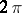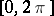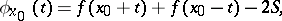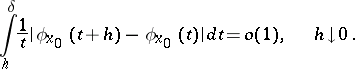# Lebesgue criterion

(diff) ← Older revision | Latest revision (diff) | Newer revision → (diff)

A criterion for pointwise convergence of Fourier series. If a-periodic function, integrable on the interval, satisfies the condition(*)

at a pointfor some, wherethen the Fourier series ofatconverges to the number. The criterion was proved by H. Lebesgue . Condition (*) is equivalent to the aggregate of the two conditionsThe Lebesgue criterion is more powerful then the Dirichlet criterion (convergence of series); the Jordan criterion; the Dini criterion; the de la Vallée-Poussin criterion; and the Young criterion.

How to Cite This Entry:
Lebesgue criterion. Encyclopedia of Mathematics. URL: http://encyclopediaofmath.org/index.php?title=Lebesgue_criterion&oldid=17261
This article was adapted from an original article by B.I. Golubov (originator), which appeared in Encyclopedia of Mathematics - ISBN 1402006098. See original article